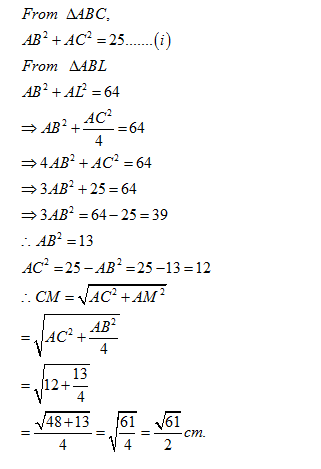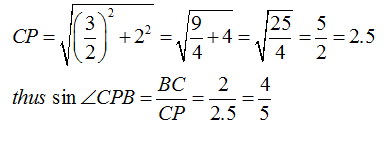• This is an assessment test.
• These tests focus on geometry and mensuration and are meant to indicate your preparation level for the subject.
• Kindly take the tests in this series with a pre-defined schedule.

## Geometry and Mensuration: Test 25

Congratulations - you have completed Geometry and Mensuration: Test 25. You scored %%SCORE%% out of %%TOTAL%%. You correct answer percentage: %%PERCENTAGE%% . Your performance has been rated as %%RATING%%
 Question 1
R and S are two points on the sides PT and QT respectively of ΔPQR such that RS= 18 cm, TS= 5 cm and ÐRST= 90o. If tan ÐPQR= 3.6, then PT: TR =
 A QT: 2 TS B 2 TS: QT C 2 QT: TS D TS: 2 QT
Question 1 Explanation:$\displaystyle \begin{array}{l}\angle \,RST={{90}^{o}}\\RS=18\,cm\\TS=5\,cm\\\therefore \,\,\tan \,T=\frac{RS}{TS}=\frac{18}{5}=3.6\\\tan \,\angle PQR=3.6\\\therefore \angle T=\angle Q\\\therefore PT=PQ\\\angle T+\angle R={{90}^{o}}\\\Rightarrow 2\,\angle T+2\,\angle R={{180}^{o}}\\\angle T+\angle P+\angle Q={{180}^{o}}\\\Rightarrow \,2T+\angle P={{180}^{o}}\\\therefore \,\,\angle P=2\,\angle R\\\therefore \,\frac{PT}{TQ}=\frac{2TR}{TS}\end{array}$
 Question 2
BL and CM are medians of ΔABC right-angled at A and BC= 5cm. If BL = 8cm, then the length of CM is
 A √61/2cm B √48/2cm C 10√2cm D 4 cm
Question 2 Explanation:Question 3
ABCD is a rectangle where the ratio of the lengths of AB and BC is 3: 2. If P is the mid-point of AB, then the value of sin ∠CPB is
 A 3/5 B 2/5 C 3/4 D 4/5
Question 3 Explanation:Question 4
PQRS is a square, T is the mid-point of PQ and M is the mid-point of QR. PM and ST are joined and they meet at L. Then which of the following is correct?
 A TP: TL= 1: 2 B PM = TS C ∠PMS = ∠PMQ D ∠PTS = ∠QPM
Question 4 Explanation:$\displaystyle \begin{array}{l}If\,\,\,PQ=2x,\,\,then\,\,QM=x\\\therefore \,\,PM=\sqrt{4{{x}^{2}}+{{x}^{2}}}=\sqrt{5}x\\Similarly,\\TS=\sqrt{4{{x}^{2}}+{{x}^{2}}}=\sqrt{5}x\end{array}$
Hence PM=TS
 Question 5
If the sums of the interior angles of a regular polygon be 1080o, the number of sides of the polygon is
 A 6 B 8 C 10 D 12
Question 5 Explanation:
Let the number of sides be x.
180(x-2)= 1080,
=> x - 2 = 6=> x = 8
Thus the correct option is (b)
Once you are finished, click the button below. Any items you have not completed will be marked incorrect.
There are 5 questions to complete.
 ← List →